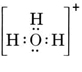# 5.5: Polyatomic Ions

$$\newcommand{\vecs}{\overset { \rightharpoonup} {\mathbf{#1}} }$$ $$\newcommand{\vecd}{\overset{-\!-\!\rightharpoonup}{\vphantom{a}\smash {#1}}}$$$$\newcommand{\id}{\mathrm{id}}$$ $$\newcommand{\Span}{\mathrm{span}}$$ $$\newcommand{\kernel}{\mathrm{null}\,}$$ $$\newcommand{\range}{\mathrm{range}\,}$$ $$\newcommand{\RealPart}{\mathrm{Re}}$$ $$\newcommand{\ImaginaryPart}{\mathrm{Im}}$$ $$\newcommand{\Argument}{\mathrm{Arg}}$$ $$\newcommand{\norm}{\| #1 \|}$$ $$\newcommand{\inner}{\langle #1, #2 \rangle}$$ $$\newcommand{\Span}{\mathrm{span}}$$ $$\newcommand{\id}{\mathrm{id}}$$ $$\newcommand{\Span}{\mathrm{span}}$$ $$\newcommand{\kernel}{\mathrm{null}\,}$$ $$\newcommand{\range}{\mathrm{range}\,}$$ $$\newcommand{\RealPart}{\mathrm{Re}}$$ $$\newcommand{\ImaginaryPart}{\mathrm{Im}}$$ $$\newcommand{\Argument}{\mathrm{Arg}}$$ $$\newcommand{\norm}{\| #1 \|}$$ $$\newcommand{\inner}{\langle #1, #2 \rangle}$$ $$\newcommand{\Span}{\mathrm{span}}$$

Our discussion of ionic compounds was confined to monatomic ions. However, more complex ions, containing several atoms covalently bonded to one another, but having a positive or negative charge, occur quite frequently in chemistry. The charge arises because the total number of valence electrons from the atoms cannot produce a stable structure. With one or more electrons added or removed, a stable structure results. Well-known examples of such polyatomic ions are the sulfate ion (SO42–),

the hydroxide ion (OH),

the hydronium ion (H3O+),

and the ammonium ion (NH4+).

The atoms in these ions are joined together by covalent electron-pair bonds, and we can draw Lewis structures for the ions just as we can for molecules. The only difference is that the number of electrons in the ion does not exactly balance the sum of the nuclear charges. Either there are too many electrons, in which case we have an anion, or too few, in which case we have a cation.

Consider, for example, the hydroxide ion (OH) for which the Lewis structure isA neutral molecule containing one O and one H atom would contain only seven electrons, six from O and one from H. The hydroxide ion, though, contains an octet of electrons, one more than the neutral molecule. The hydroxide ion must thus carry a single negative charge. In order to draw the Lewis structure for a given ion, we must first determine how many valence electrons are involved. Suppose the structure of H3O+ is required. The total number of electrons is obtained by adding the valence electrons for each atom, 6 + 1 + 1 + 1 = 9 electrons. We must now subtract 1 electron since the species under consideration is not H3O but H3O+. The total number of electrons is thus 9 – 1 = 8. Since this is an octet of electrons, we can place them all around the O atom. The final structure then follows very easily:In more complicated cases it is often useful to calculate the number of shared electron pairs before drawing a Lewis structure. This is particularly true when the ion in question is an oxyanion (i.e., a central atom is surrounded by several O atoms). A well-known oxyanion is the carbonate ion, which has the formula CO32–. (Note that the central atom C is written first, as was done earlier for molecules.) The total number of valence electrons available in CO32– is

$$4 \text{(for C)} + 3 \times 6 \text{(for O)} + 2 \text{(for the –2 charge)} = 24$$

We must distribute these electrons over 4 atoms, giving each an octet, a requirement of 4 × 8 = 32 electrons. This means that 32 – 24 = 8 electrons need to he counted twice for octet purposes; i.e., 8 electrons are shared. The a ion thus contains four electron-pair bonds. Presumably the C atom is double-bonded to one of the O’s and singly bonded to the other two:In this diagram the 4C electrons have been represented by dots, the 18 O electrons by ×’s, and the 2 extra electrons by colored dots, for purposes of easy reference. Real electrons do not carry labels like this; they are all the same.

There is a serious objection to the Lewis structure just drawn. How do the electrons know which oxygen atom to single out and form a double bond with, since there is otherwise nothing to differentiate the oxygens? The answer is that they do not. To explain the bonding in the CO32– ion and some other molecules requires an extension of the Lewis theory. We pursue this matter further when we discuss resonance. Now we end with an example.

Example $$\PageIndex{1}$$ : Lewis Structure

Draw a Lewis structure for the sulfite ion, SO32–.

Solution The safest method here is to count electrons. The total number of valence electrons available is

6(for S) + 3 × 6(for O) + 2(for the charge) = 26 To make four octets for the four atoms would require 32 electrons, and so the difference, 32 – 26 = 6, gives the number of shared electrons. There are thus only three electron-pair bonds in the ion. The central S atom must be linked by a single bond to each O atom.Note that each of the S—O bonds is coordinate covalent.